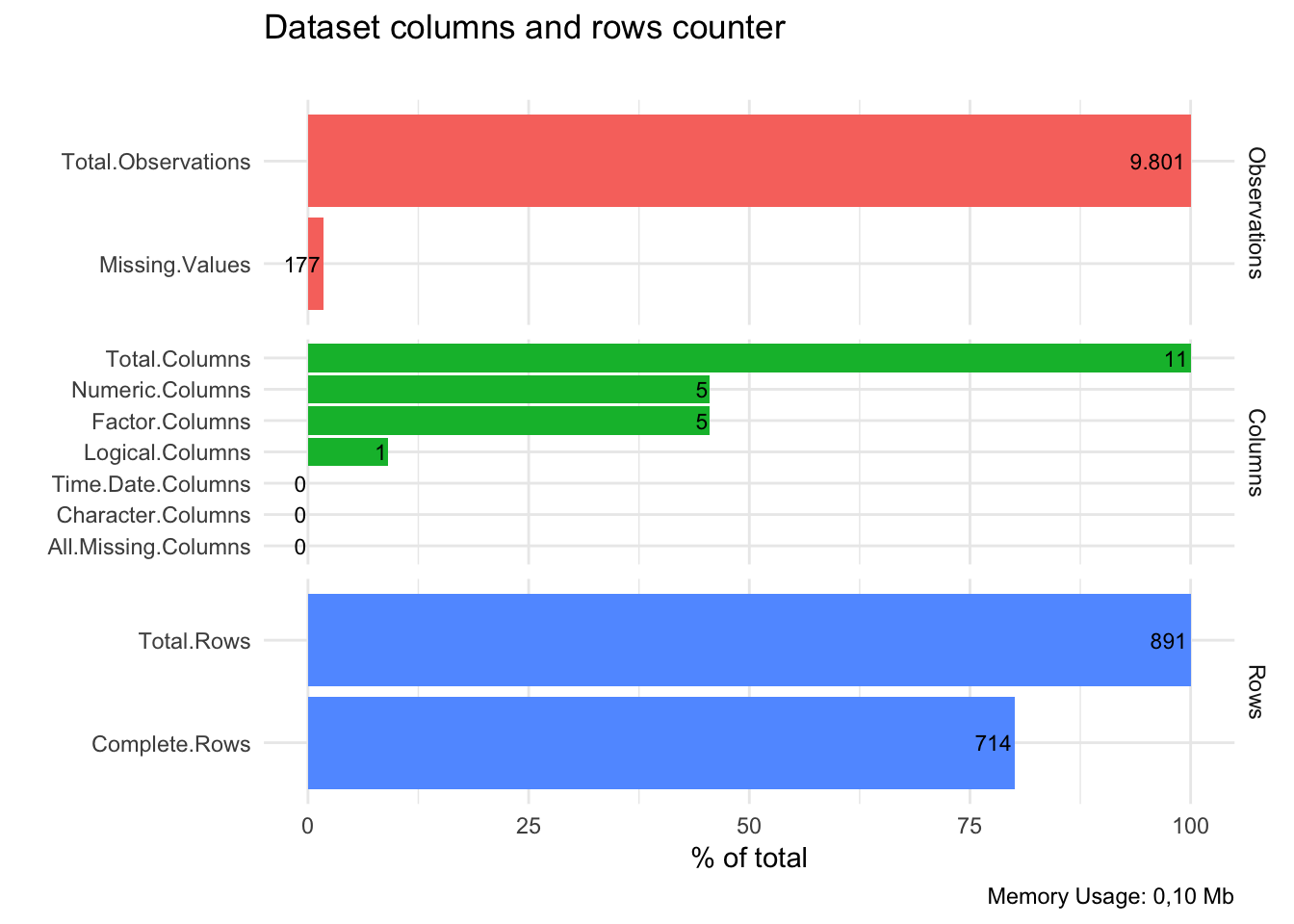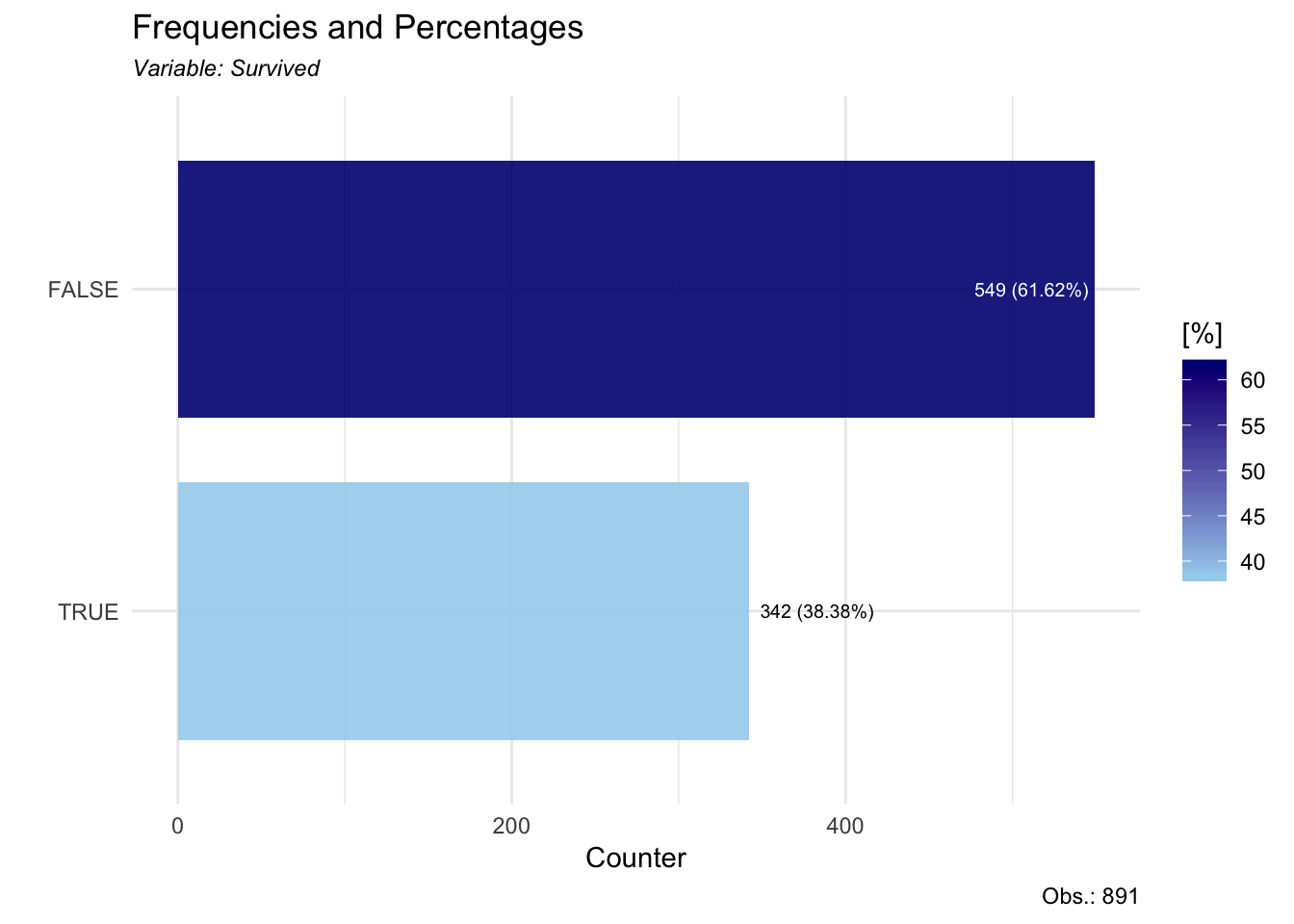This is a quick tutorial on using the most useful functions in the `lares` library for quick data analysis: `freqs`, `distr`, and `corr_var`. With these you can explore and understand the interaction between all or specific variables within a dataset.

First, we load the `lares` and `tidyverse` libraries (for `dplyr` and `ggplot2`)

``````library(lares) # devtools::install_github("laresbernardo/lares")
library(tidyverse)``````

Then, we load the `dft` dataset within the `lares` library. It is a useful subset from the Titanic survival dataset.

``data(dft)``

Let’s quickly see its structure:

``df_str(dft, return = "plot")````head(dft, 5)``

## FREQUENCIES (freqs):

This function lets us group, count, calculate percentages and cumulatives for further transformations or for visual analysis. For more details: `?freqs`

``````# How many survived?
dft %>% freqs(Survived)``````
``````# How many survived and see plot?
dft %>% freqs(Survived, plot = T, results = F)````````````# How many survived per class?
dft %>% freqs(Survived, Pclass, plot = T, results = F)``````